# Mixing Dry Chemicals

## Total Chemical Application Weight

This calculator finds how much dry chemical to mix with water.

Area that the Chemical is Applied to:
Application Rate:
Mix Concentration (concentration of desired chemical in dry media):
%
Dry Chemical Weight:

## Minimum Water Volume for Mixing Dry Chemicals

This calculates the minimum amount of water that must be mixed with dry chemicals in order to get the chemicals dissolved into solution. Adding more water than the minimum is necessary.

Weight of Dry Chemical to be Dissolved:
Chemical Solubility (from the label):

## The Equations

To calculate the weight of dry chemical needed use this formula.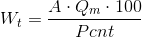Where: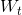= The mass of the chemical to be applied in pounds (lbs).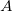= The area in acres (acres).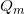= Rate to apply by pounds per acre (lbs/acre)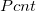= Percent concentration in the mix.

The equation to determine the minimum amount of waterthat must be added to completely disolve the dry chemicals is: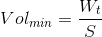Where:= Weight of the chemical in the tank in pounds.= Solubility of the chemical in pounds per gallon.

WSU Prosser - IAREC, 24106 N Bunn Rd, Prosser WA 99350-8694, 509-786-2226,Contact Us